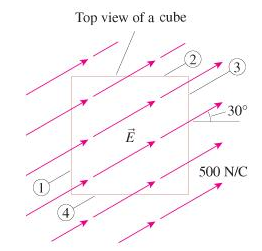# Problem: The figure(Figure 1) shows four sides of a 6.0cm×6.0cm×6.0cm cube.(a) What is the electric flux Φ1 through side 1?(b) What is the electric flux Φ2 through side 2?(c) What is the electric flux Φ3 through side 3?(d) What is the electric fluxe Φ4 through side 4?

###### FREE Expert Solution

Electrix flux:

$\overline{){{\mathbf{\varphi }}}_{{\mathbf{E}}}{\mathbf{=}}{\mathbf{E}}{\mathbf{A}}{\mathbf{c}}{\mathbf{o}}{\mathbf{s}}{\mathbf{\theta }}}$

Area of a side of the cube = 0.06 × 0.06 = 0.0036m2

(a)

The angle between side one and the electric field is 150°

Φ1 = EAcosθ = (500)(0.0036)cos150 = -1.56N•m2/C

79% (209 ratings)###### Problem Details

The figure(Figure 1) shows four sides of a 6.0cm×6.0cm×6.0cm cube.(a) What is the electric flux Φ1 through side 1?

(b) What is the electric flux Φ2 through side 2?

(c) What is the electric flux Φ3 through side 3?

(d) What is the electric fluxe Φ4 through side 4?